Next: RESAMPLE Up: COREGPM Previous: Output Description   Contents

# Implementation

The observation equations are given by the polynomial model (y = A x):(T2)

Where:
y contains the observed offsets in a certain direction.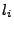denotes the location (line number) of the observed offsets in a certain direction.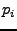denotes the location (pixel number) of the observed offsets in a certain direction.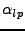denotes the unknown coefficients of the polynomial.

The data is rescaled (to the interval [-2, 2], see Annex D) so the normalmatrix is rescaled. otherwise there could occur very high values for, e.g.,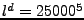. The least squares parameter solution is given by: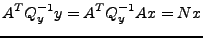(T3)

Where: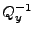is the (diagonal) covariance matrix of the observations. this matrix can be equal to identity or to the correlation values in version 1. (CPM_WEIGHT card).

The coefficients are estimated by factorization of the matrix N.

The inverse of matrix N is also computed to check the solution (stability) and to compute some statistics.

A check number is given (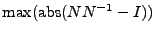) that gives a hint on the stability of the solution.Next: RESAMPLE Up: COREGPM Previous: Output Description   Contents
Leijen 2009-04-14